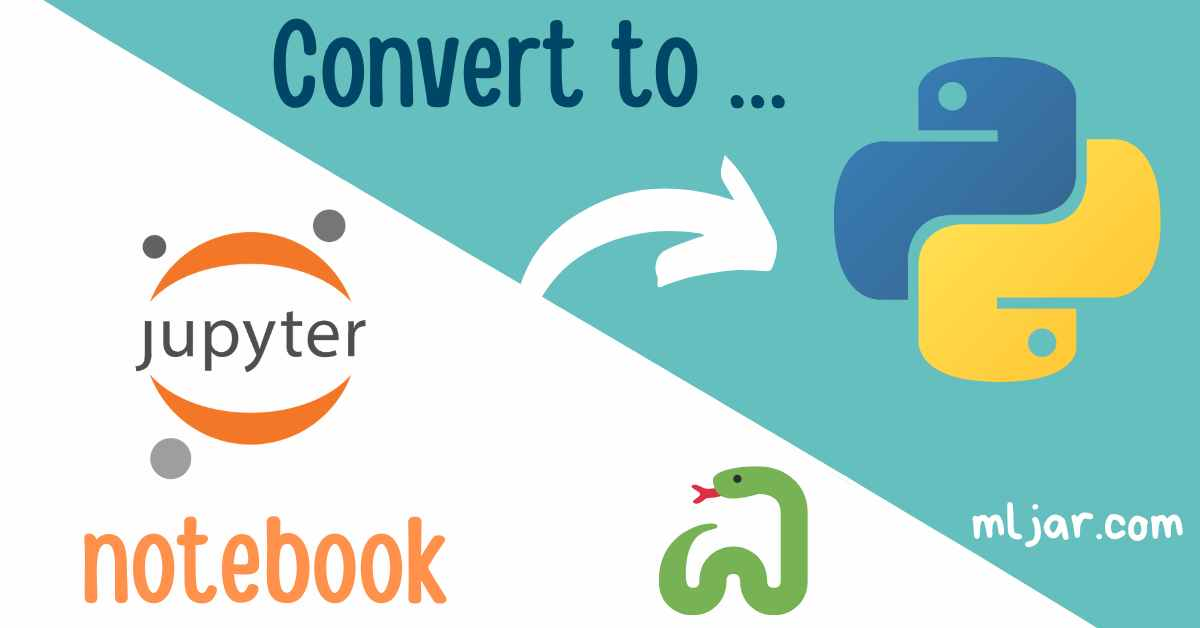Jupyter Notebook saves files in `.ipynb` format. It is a JSON with code, Markdown, and outputs. There are many cases in which we would like to convert Jupyter Notebook to plain Python script. For example, you would like to keep Python code in the repository or would like to turn your notebook into a standalone package. I will show you 3 ways to export the Jupyter Notebook file to Python script.

Firstly, let’s create an example notebook. It has Markdown mixed with Python code. Figure and Pandas DataFrame are outputs. The notebook is presented in the image below: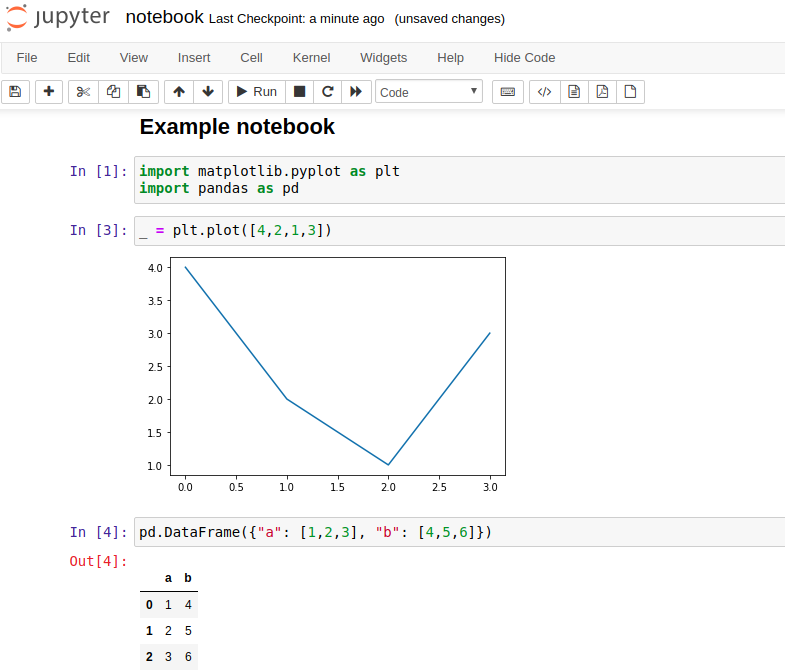The notebook is saved as a `.ipynb` file. Below is the content of the `notebook.ipynb` file.

``````{
"cells": [
{
"cell_type": "markdown",
"id": "7ca6b82b",
"source": [
"## Example notebook"
]
},
{
"cell_type": "code",
"execution_count": 1,
"id": "73f3924a",
"outputs": [],
"source": [
"import matplotlib.pyplot as plt\n",
"import pandas as pd"
]
},
{
"cell_type": "code",
"execution_count": 3,
"id": "3a691d7f",
"outputs": [
{
"data": {
"image/png": "iVBORw0KGgoA ...

... +bwAAAABJRU5ErkJggg==\n",
"text/plain": [
"<Figure size 432x288 with 1 Axes>"
]
},
"needs_background": "light"
},
"output_type": "display_data"
}
],
"source": [
"_ = plt.plot([4,2,1,3])"
]
},
{
"cell_type": "code",
"execution_count": 4,
"id": "934cc473",
"outputs": [
{
"data": {
"text/html": [
"<div>\n",
"<style scoped>\n",
"    .dataframe tbody tr th:only-of-type {\n",
"        vertical-align: middle;\n",
"    }\n",
"\n",
"    .dataframe tbody tr th {\n",
"        vertical-align: top;\n",
"    }\n",
"\n",
"        text-align: right;\n",
"    }\n",
"</style>\n",
"<table border=\"1\" class=\"dataframe\">\n",
"    <tr style=\"text-align: right;\">\n",
"      <th></th>\n",
"      <th>a</th>\n",
"      <th>b</th>\n",
"    </tr>\n",
"  <tbody>\n",
"    <tr>\n",
"      <th>0</th>\n",
"      <td>1</td>\n",
"      <td>4</td>\n",
"    </tr>\n",
"    <tr>\n",
"      <th>1</th>\n",
"      <td>2</td>\n",
"      <td>5</td>\n",
"    </tr>\n",
"    <tr>\n",
"      <th>2</th>\n",
"      <td>3</td>\n",
"      <td>6</td>\n",
"    </tr>\n",
"  </tbody>\n",
"</table>\n",
"</div>"
],
"text/plain": [
"   a  b\n",
"0  1  4\n",
"1  2  5\n",
"2  3  6"
]
},
"execution_count": 4,
"output_type": "execute_result"
}
],
"source": [
"pd.DataFrame({\"a\": [1,2,3], \"b\": [4,5,6]})"
]
},
{
"cell_type": "code",
"execution_count": null,
"id": "17200066",
"outputs": [],
"source": []
}
],
"kernelspec": {
"display_name": "pystokenv",
"language": "python",
"name": "pystokenv"
},
"language_info": {
"codemirror_mode": {
"name": "ipython",
"version": 3
},
"file_extension": ".py",
"mimetype": "text/x-python",
"name": "python",
"nbconvert_exporter": "python",
"pygments_lexer": "ipython3",
"version": "3.8.0"
}
},
"nbformat": 4,
"nbformat_minor": 5
}

``````

The notebook is a collection of cells. Each cell has information about the source and outputs (with several formats). Images are encoded with Base64.

We can use the User Interface provided in the Jupyter Notebook to export the notebook to Python `.py` file. Please click `File`->`Download as`->`Python (.py)`.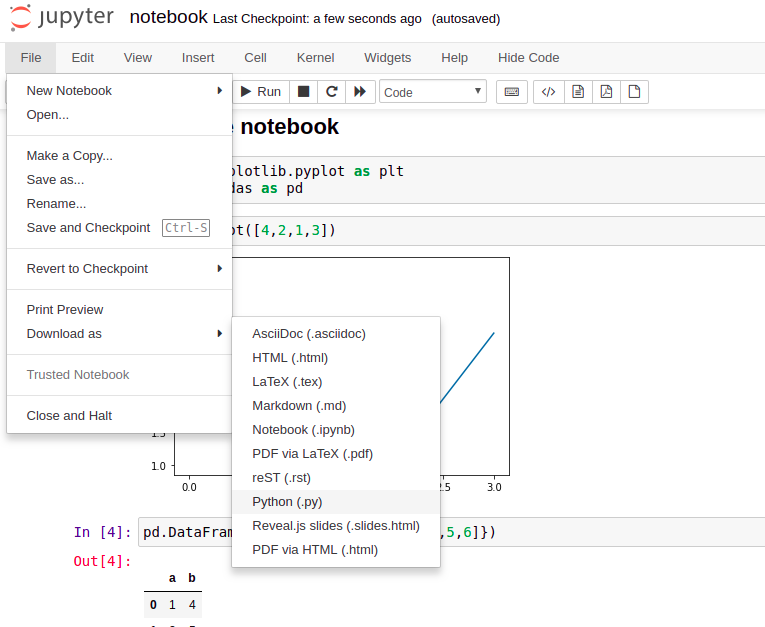The file `notebook.py` will be downloaded from the web browser, its content is below:

``````#!/usr/bin/env python
# coding: utf-8

# ## Example notebook

# In:

import matplotlib.pyplot as plt
import pandas as pd

# In:

_ = plt.plot([4,2,1,3])

# In:

pd.DataFrame({"a": [1,2,3], "b": [4,5,6]})

# In[ ]:

``````

Only Markdown and Python code is included in the Python script. Outputs are omitted. There are added comments with information about cells: `# In`. The Markdown text is commented on. The shebang for Python is added to the file beginning.

## 2. Convert Jupyter Notebook to Python in the command line

You can export your notebook to the `.py` file using the command line. There is a `nbconvert` tool available. It is installed with Jupyter Notebook because it is used internally (in the `Download as` functionality).

``````jupyter nbconvert --to python notebook.ipynb
``````

Example output:

``````[NbConvertApp] Converting notebook notebook.ipynb to python
[NbConvertApp] Writing 233 bytes to notebook.py
``````

The resulting Python file has the same content as created with User Interface.

## 3. Use `jupytext` to pair the notebook with the Python script

There is a `jupytext` package that can be used to synchronize the Jupyter Notebook file with a plain Python file - you will have the same code in both files `.pynb` and `.py`. What is more, there is a command line version available.

You can install `jupytext` with the below commands:

``````pip install jupytext

# or

conda install jupytext -c conda-forge
``````

After installation, there will be a new menu available under `File`. You can select how your notebook should be paired with the Python script: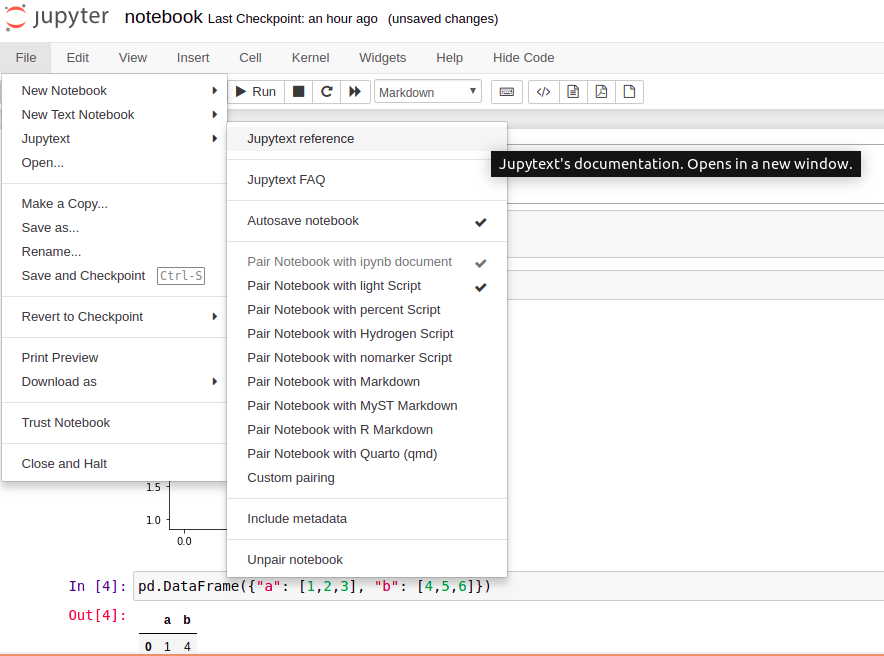The Python script from the example notebook in a light format is presented below:

``````# ## Example notebook

import matplotlib.pyplot as plt
import pandas as pd

_ = plt.plot([4,2,1,3])

pd.DataFrame({"a": [1,2,3], "b": [4,5,6]})

``````

The same script in a percent format is below. Cells are delimited with `# %%` This format is supported by many IDEs (VS Code, Spyder, PyCharm).

``````# %% [markdown]
# ## Example notebook

# %%
import matplotlib.pyplot as plt
import pandas as pd

# %%
_ = plt.plot([4,2,1,3])

# %%
pd.DataFrame({"a": [1,2,3], "b": [4,5,6]})

# %%

``````

The Python script will be updated whenever you will save a notebook file.

You can obtain the Python script from a notebook with the command line:

``````# convert to Python script with light format
jupytext --to py notebook.ipynb

# convert to Python script with percent format
jupytext --to py:percent notebook.ipynb
``````

The `jupytext` package works well with Python, Julia, and R. You can read more about its options in their documentation.

## Summary

The Jupyter Notebook is a great place to explore with code and share with others. It uses the `.ipynb` file to store code, Markdown, and outputs as JSON. Many times we will need to export the notebook to plain Python script. It can be done using the User Interface or command line. There are two packages worth remembering: `nbconvert` and `jupytext`.

Just a side note, if you would like to schedule Jupyter Notebook for periodic execution, you don’t need to convert it to Python script. You can schedule the automatic execution of the notebook; please check our article about Jupyter Notebook scheduling.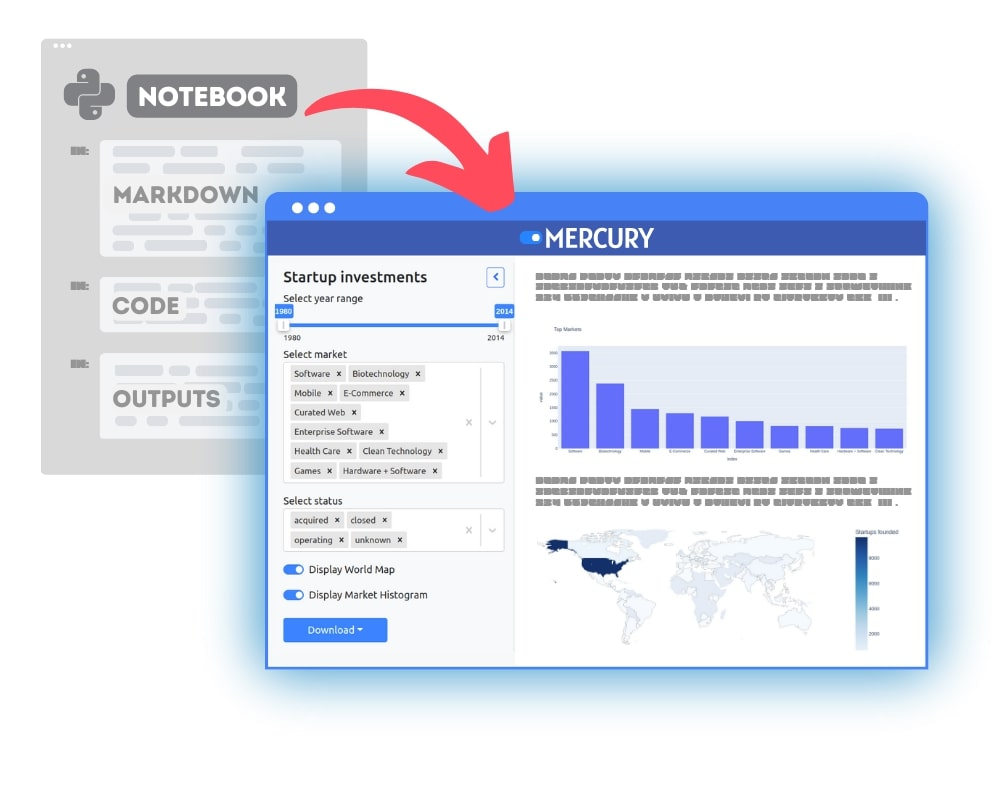### Convert Python Notebooks to Web Apps

We are working on open-source framework Mercury for converting Jupyter Notebooks to interactive Web Applications.### Articles you might find interesing